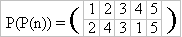# Permutations

Time Limit: 1000MS

Memory Limit: 65536K

## Description

We remind that the permutation of some final set is a one-to-one mapping of the set onto itself. Less formally, that is a way to reorder elements of the set. For example, one can define a permutation of the set {1,2,3,4,5} as follows:This record defines a permutation P as follows: P(1) = 4, P(2) = 1, P(3) = 5, etc. What is the value of the expression P(P(1))? It’s clear, that P(P(1)) = P(4) = 2. And P(P(3)) = P(5) = 3. One can easily see that if P(n) is a permutation then P(P(n)) is a permutation as well. In our example (believe us)It is natural to denote this permutation by P2(n) = P(P(n)). In a general form the defenition is as follows: P(n) = P1(n), Pk(n) = P(Pk-1(n)). Among the permutations there is a very important one — that moves nothing:It is clear that for every k the following relation is satisfied: (EN)k = EN. The following less trivial statement is correct (we won't prove it here, you may prove it yourself incidentally): Let P(n) be some permutation of an N elements set. Then there exists a natural number k, that Pk = EN. The least natural k such that Pk = EN is called an order of the permutation P. The problem that your program should solve is formulated now in a very simple manner: "Given a permutation find its order."

## Input

In the first line of the standard input an only natural number N (1 <= N <= 1000) is contained, that is a number of elements in the set that is rearranged by this permutation. In the second line there are N natural numbers of the range from 1 up to N, separated by a space, that define a permutation — the numbers P(1), P(2),…, P(N).

## Output

You should write an only natural number to the standard output, that is an order of the permutation. You may consider that an answer shouldn't exceed 109.

## Sample Input

5
4 1 5 2 3


## Sample Output

6

N/A